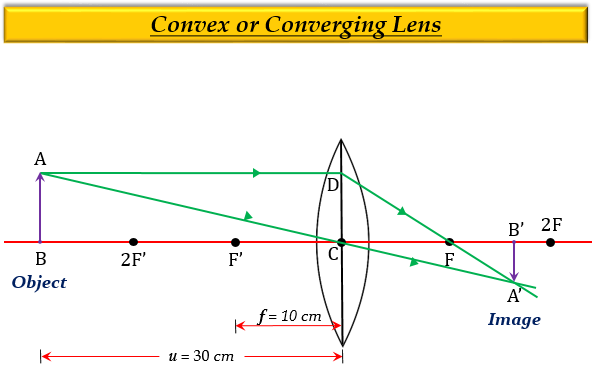# One half of a convex lens of focal length 10 cm is covered with black paper. Can such a lens produce an image of a complete object placed at a distance of 30 cm from the lens? Draw a ray diagram to justify your answer. A 4 cm tall object is placed perpendicular to its principal axis of a convex lens of focal length 20 cm. The distance of the object from the lens is 15 cm. Find the nature, position, and size of the image.

One-half of a convex lens of focal length 10 cm is covered with black paper. Yes, such a lens can produce an image of a complete object placed at a distance of 30 cm from the lens. Because the light coming from the object can be refracted from the other half of the lens. The image formed will be real, inverted, and diminished.

The ray diagram for the justification of the answer is as follows:Given:

Object size, $h=+4cm$

Object distance, $u=–15cm$

Focal length, $f=+20cm$

To find: Image distance, or position $v$, nature and size of the image, $h'$.

Solution:

From the lens formula, we know that-

$\frac {1}{f}=\frac {1}{v}-\frac {1}{u}$

Substituting the required values, we get-

$\frac {1}{20}=\frac {1}{v}-\frac {1}{(-15)}$

$\frac {1}{20}=\frac {1}{v}+\frac {1}{15}$

$\frac {1}{v}=\frac {1}{20}-\frac {1}{15}$

$\frac {1}{v}=\frac {3-4}{60}$

$\frac {1}{v}=-\frac {1}{60}$

$v=-60cm$

Thus the screen image distance or position, $v$ is 60 cm from the lens, and the minus sign implies that the image forms in front of the lens (on the left side). Also, the image will be virtual as it forms on the left side of the lens.

Now,

From the magnification formula, we know that-

$m=\frac {v}{u}=\frac {h'}{h}$

Substituting the required values, we get-

$\frac {-60}{-15}=\frac {h'}{4}$

$4=\frac {h'}{4}$

$h'=+16cm$

Thus, the height or size of the image, $h'$ is 16 cm, and the plus sign implies that the image forms erect (upright ).

Therefore, the image formed will be virtual, erect, and magnified.

Updated on: 10-Oct-2022

4K+ Views